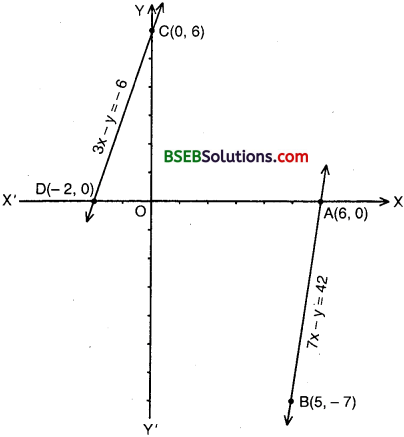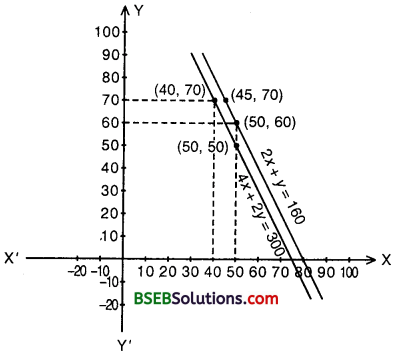# Bihar Board Class 10th Maths Solutions Chapter 3 Pair of Linear Equations in Two Variables Ex 3.1

Bihar Board Class 10th Maths Solutions Chapter 3 Pair of Linear Equations in Two Variables Ex 3.1 Textbook Questions and Answers.

## BSEB Bihar Board Class 10th Maths Solutions Chapter 3 Pair of Linear Equations in Two Variables Ex 3.1

Question 1.
Aftab tells his daughter, “Seven years ago, I was seven times as old as you were then. Also, three years from now, I shall be three times as old as you will be”. (Isn’t this interesting)? Represent this situation algebraically and graphically.
Solution:
Let us denote the present age of daughter and her father Aftab as x years and y years respectively. Then, algebraic representation is given by the following equations:
7(x – 7) = y – 7 or 7x – 49 = y – 7
or 7x – y = 42 ………………. (1)
and 3(x + 3) = y + 3 or 3x + 9 = y + 3
or 3x – y = – 6 ……………….. (2)
To obtain the equivalent graphical representation, we find two points on the line representing each equation. That is, we find two solutions of each equation. These solutions are given below in the tables:
For 7x – y = 42For 3x – y = – 6To represent these equations graphically, we plot the points A(6, 0) and B(5, – 7) to get the graph of (1) and the points C(0, 6) and D(- 2, 0) give the graph of (2).Question 2.
The coach of a cricket team buys 3 bats and 6 balls for Rs 3900. Later, she buys another bat and 2 more balls of the same kind for Rs 1300. Represent this situation algebraically and geometrically.
Solution:
Let us denote the cost of one bat by Rs x and one ball by Rs y. Then, the algebraic representation is given by the following equations:
3x + 6y = 3900 ……………… (1)
and x + 2y = 1300 …………….. (2)
To obtain the equivalent geometric representation, we find two points on the line representing each equation. That is, we find two solutions of each equation. These solutions are given below in the table:We plot the points A(0, 650) and B(1300, 0) to obtain the geometric representation of 3x + 6y = 3900 and C(500, 400) and D(100, 600) to obtain the geometric representation of x + 2y = 1300.We observe that these lines are coincident.Question 3.
The cost of 2 kg of apples and 1 kg of grapes on a day was found to be Rs 160. After a month, the cost of 4 kg of apples and 2 kg of grapes is Rs 300. Represent the situation algebraically and geometrically.
Solution:
Let us denote the cost of 1 kg of apples by Rs x and cost of 1 kg of grapes by Rs y.
Then the algebraic representation is given by the following equations:
2x + y = 160 …………….. (1)
4x + 2y = 300 ……………….. (2)
To find the equivalent geometric representation, we find two points on the line representing each equation. That is, we find two solutions of each equation.Geometric representation is shown on the previous pages which is a pair of parallel lines.